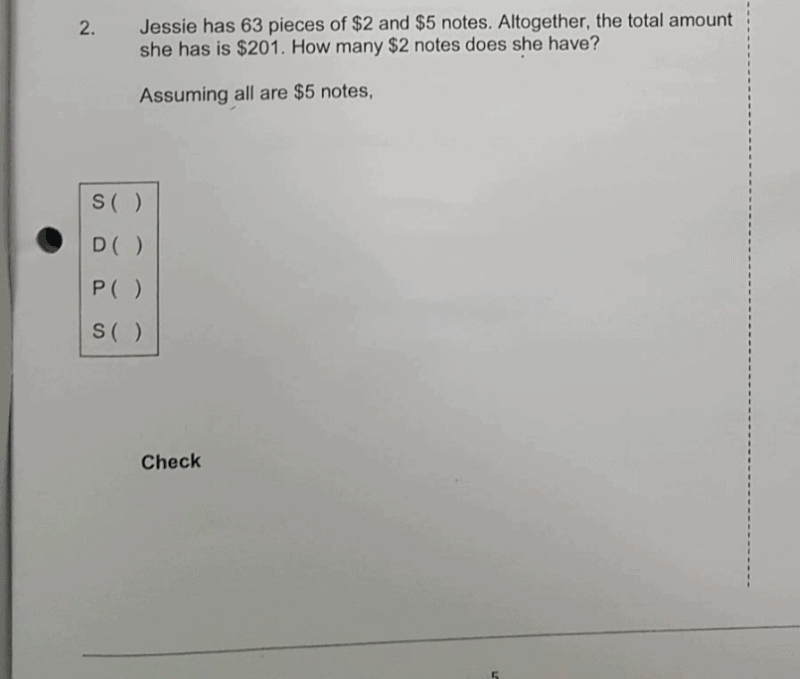# QuestionHi any one can help thks

Source: 2017 sa2

The original question ended with “Assume all are \$5 notes,” so I assume the working is expected to start with that. So here goes:

```Assume all are \$5 notes, so total is 63 x \$5 = \$315.
There is extra \$315 - \$201 = \$114.Replace a \$5 with a \$2 and the total drops by \$3.
Now 114/3 = 38. So if we turn 38 \$5 notes into \$2,
we drop by \$114 and total becomes \$201.
So there must be 38 \$2 notes.

CHECK:
\$2 notes: 38
\$5 notes: 63 - 38 = 25
Value = 25 x \$5 + 38 x \$2 = \$201```

Ehh I wouldn’t do guess and check. It sucks a lot and I would cry a ton drawing the darn tables. There’s a way better method and that is the assumption method. So I don’t have the time to explain as it is way past my bedtime but I’ll show you the solution :

Total (or maximum you can get in the \$5 notes) : 63 pieces * 2 = \$126.

Extra (Meaning the total/maximum minus what Jessie has, or you may flip the roles) :

\$201 (Jessie’s money) – \$126 (Total for \$2 notes) = \$75

Difference (for each quantity per unit, in this case, one five-dollar note minus two-dollar note) :

Simple. \$5 – \$2 = \$3.

Opposite (Magic step. This is changing from one thing to another by dividing Extra and Difference):

75 / 3 = 25

So there are 25 \$2 notes.

Note: Yeah I knew it, I checked this answer then realized I got it wrong. Yep, I should’ve done 63 * 5 instead of 2. Now I look stupid. Btw new answer = 38.

This is a P4 question. Learning guess and check first will help the student to appreciate the supposition and assumption method. After all the suppostion and assumptioin method is derived from the guess and check method.

0 Replies 0 Likes

 Number of \$2 notes Number of \$5 noted Value of \$2 notes Value of \$5 notes Total value 0 63 0 315 315 1 62 2 310 312 2 61 4 305 309 ? (how many decremental to get to here?) 201

What’s the decremental of the total value with each iteration? It’s 3.  for exmple, 315 – 312 = 3, so is 312 – 309.

### Number of decrementals = (315 – 201)/3 = 38

And this correspond to the number of \$2 notes.  So the answer is 38.

You will learn the supposition and assuption method maybe in P5 or P6, you will see that it is derived from this simple guess and check method.

Assumption and Suppsition, will have a lot less to write in term of workings.

Assume all are \$5 notes. Total value is \$315

Difference between one \$5 note and one \$2 notes = \$3 (this is the decremental in the guess and check above)

Number of of \$2 notes = (315-210)/3 = 38 (notice this is exactly like the calculation above for the number of decrementals.)

0 Replies 1 Like